### Home > MC2 > Chapter 6 > Lesson 6.2.6 > Problem6-128

6-128.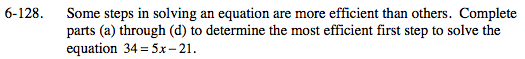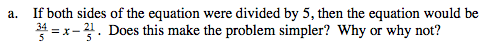Fractional coefficients are more complex and can be harder to deal with than whole number coefficients.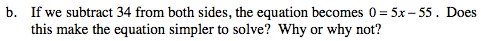All terms are on one side of the equation.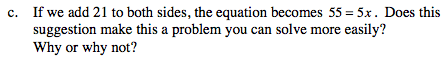The variable x has been isolated on one side of the equation. What would you do next to solve the equation?
Is this one less step than you would take for the other first steps from parts (a) and (b)?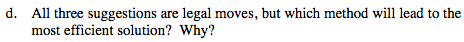Part (c) is the most efficient method. Can you see why?
Try using each method to see which is the quickest with the least steps to solve the equation.